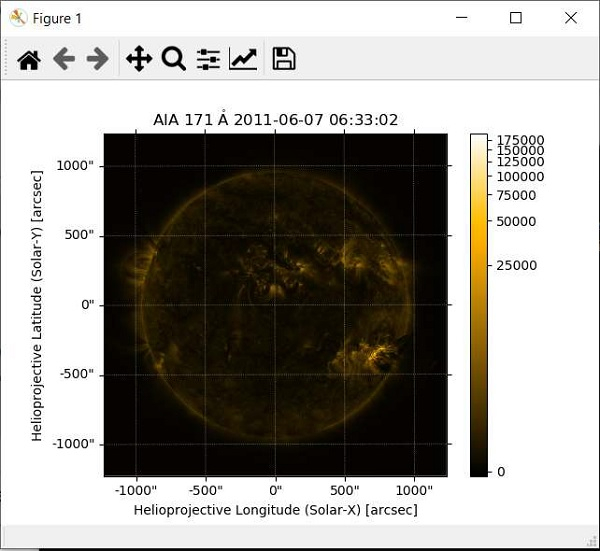# SunPy Plotting a Solar Image in Python

In this tutorial, we are going to learn how to plot a solar image using SunPy module.

Let's install the module using the following command.

pip install sunpy

Now, we have the module. Before going into writing the actual code, we have to download the data related to sample data for plotting the image. We can download the sample data using the following code.

## Example

# importing the data
import sunpy.data
sunpy.data.download_sample_data()

## Example

# importing the image from sample data
from sunpy.data.sample import AIA_171_IMAGE
# importing the map for solar image
import sunpy.map
# creating the Map with sample data
solar_image = sunpy.map.Map(AIA_171_IMAGE)
# plotting the image
solar_image.peek()

## Output

If you run the above code, then you will get the image as follows.